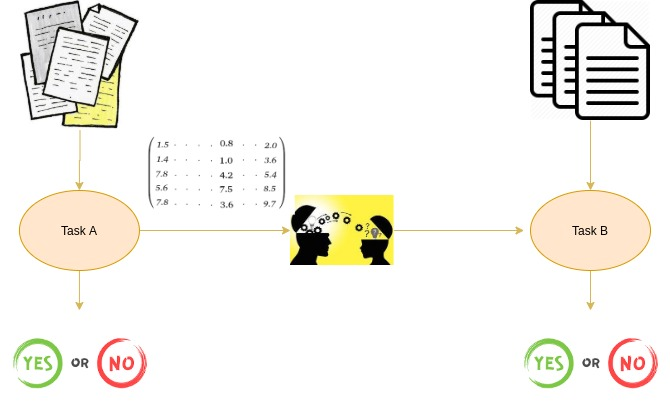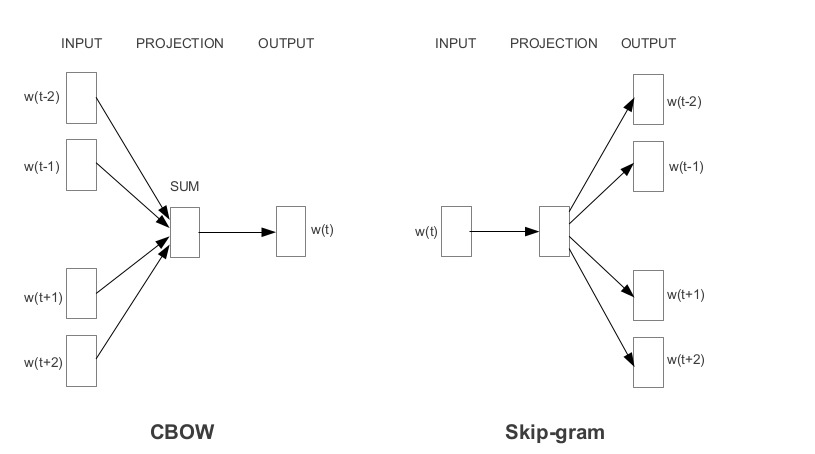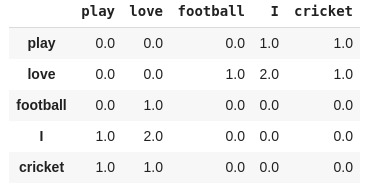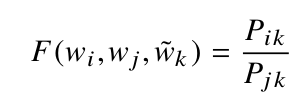# 预训练词嵌入

### 概述

• 理解预训练词嵌入的重要性

• 了解两种流行的预训练词嵌入类型：Word2Vec和GloVe

• 预训练词嵌入与从头学习嵌入的性能比较

### 介绍#### 目录

1. 什么是预训练词嵌入？

2. 为什么我们需要预训练的词嵌入？

3. 预训练词嵌入的不同模型？

1. 谷歌的Word2vec

2. 斯坦福的GloVe

4. 案例研究：从头开始学习嵌入与预训练词嵌入

### 什么是预训练词嵌入？• 训练数据稀疏

• 大量可训练参数

### 预训练词嵌入的不同模型

1. 谷歌的Word2vec

2. 斯坦福的GloVe

#### 谷歌的Word2vec

Word2Vec的架构非常简单。它是一个只有一个隐藏层的前馈神经网络。因此，它有时被称为浅层神经网络结构。

• 连续词袋模型（CBOW）

• Skip-gram 模型

• 连续词袋模型：Input=[I，have，at，times]，Output=failed

• Skip-gram 模型跳：Input = failed, Output = [I, have, at, times ]#### 斯坦福的GloVe

GloVe嵌入的基本思想是从全局统计中导出单词之间的关系。p(cricket/play)=1

p(cricket/love)=0.5

p(cricket/play) / p(cricket/love) = 2### 案例研究：从头开始学习嵌入与预训练词嵌入

#导入库
import pandas as pd
import numpy as np

#读取csv文件

#训练测试集分离
x_tr, y_tr = train['text'].values, train['label'].values
x_val, y_val = valid['text'].values, valid['label'].values

from keras.preprocessing.text import Tokenizer

tokenizer = Tokenizer()

#准备词汇表
tokenizer.fit_on_texts(list(x_tr))

#将文本转换为整数序列
x_tr_seq  = tokenizer.texts_to_sequences(x_tr)
x_val_seq = tokenizer.texts_to_sequences(x_val)

#填充以准备相同长度的序列
x_val_seq = pad_sequences(x_val_seq, maxlen=100)

size_of_vocabulary=len(tokenizer.word_index) + 1 #+1用于填充
print(size_of_vocabulary)

Output: 112204

#深度学习库
from keras.models import *
from keras.layers import *
from keras.callbacks import *

model=Sequential()

#嵌入层

#lstm层

#Global Max池化

#Dense层

#添加损失函数、度量、优化器

#添加回调
es = EarlyStopping(monitor='val_loss', mode='min', verbose=1,patience=3)
mc=ModelCheckpoint('best_model.h5', monitor='val_acc', mode='max', save_best_only=True,verbose=1)

#输出模型
print(model.summary())history = model.fit(np.array(x_tr_seq),np.array(y_tr),batch_size=128,epochs=10,validation_data=(np.array(x_val_seq),np.array(y_val)),verbose=1,callbacks=[es,mc])

#加载最佳模型

#评估
_,val_acc = model.evaluate(x_val_seq,y_val, batch_size=128)
print(val_acc)

# 将整个嵌入加载到内存中
embeddings_index = dict()
f = open('../input/glove6b/glove.6B.300d.txt')

for line in f:
values = line.split()
word = values
coefs = np.asarray(values[1:], dtype='float32')
embeddings_index[word] = coefs

f.close()
print('Loaded %s word vectors.' % len(embeddings_index))

# 为文档中的单词创建权重矩阵
embedding_matrix = np.zeros((size_of_vocabulary, 300))

for word, i in tokenizer.word_index.items():
embedding_vector = embeddings_index.get(word)
if embedding_vector is not None:
embedding_matrix[i] = embedding_vector

model=Sequential()

#嵌入层

#lstm层

#Global Max池化

#Dense层

#添加损失函数、度量、优化器

#添加回调
es = EarlyStopping(monitor='val_loss', mode='min', verbose=1,patience=3)
mc=ModelCheckpoint('best_model.h5', monitor='val_acc', mode='max', save_best_only=True,verbose=1)

#输出模型
print(model.summary())history = model.fit(np.array(x_tr_seq),np.array(y_tr),batch_size=128,epochs=10,validation_data=(np.array(x_val_seq),np.array(y_val)),verbose=1,callbacks=[es,mc])

#加载最佳模型

#评估
_,val_acc = model.evaluate(x_val_seq,y_val, batch_size=128)
print(val_acc)

### 结尾

http://panchuang.net/

sklearn机器学习中文官方文档：
http://sklearn123.com/

http://docs.panchuang.net/